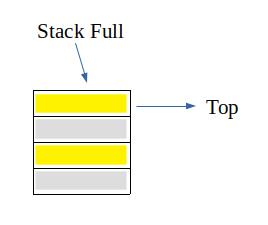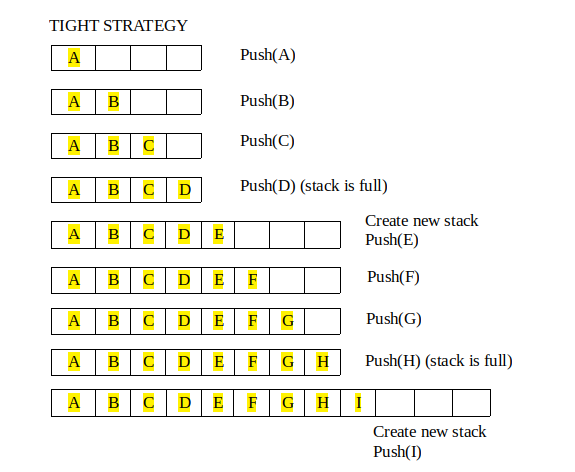Open in App
Not now

# Growable array based stack

• Difficulty Level : Easy
• Last Updated : 29 Jul, 2022

We all know about Stacks also known as Last-In-First-Out(LIFO) structures. Stack primarily has two main operation namely push and pop, where push inserts an element at top and pop removes an element from top of the stack.

Now, whenever an implementation of stack is considered its size is pre-determined or fixed. Even though it is dynamically allocated, still once it is made its size cannot be changed. And hence a condition called “stack full” arises.But what if a stack can grow as more elements are inserted or more elements are going to be inserted in future. Remember, we are talking about array based Stack. Growable Stack is the concept of allocating more memory such that “stack full” condition does not arises easily.
A Growable array-based Stack can be implemented by allocating new memory larger than previous stack memory and copying elements from old stack to new stack. And then at last change the name of new stack to the name which was given to old stack

There are two strategy for growable stack:

1.  Tight Strategy : Add a constant amount to the old stack (N+c)
2.  Growth Strategy : Double the size of old stack (2N)There are two operation on growable stack:

1.  Regular Push Operation: Add one element at top of stack
2.  Special Push Operation: Create a new stack of size greater than old stack (according to one of the strategy above) and copy all elements from old stack and then push the new element to the new stack.

Implementation:

## C++

 `// CPP Program to implement growable array based stack``// using tight strategy``#include ``using` `namespace` `std;` `// constant amount at which stack is increased``#define BOUND 4` `// top of the stack``int` `top = -1;` `// length of stack``int` `length = 0;` `// function to create new stack``int``* create_new(``int``* a)``{``    ``// allocate memory for new stack``    ``int``* new_a = ``new` `int``[length + BOUND];` `    ``// copying the content of old stack``    ``for` `(``int` `i = 0; i < length; i++)``        ``new_a[i] = a[i];` `    ``// re-sizing the length``    ``length += BOUND;``    ``return` `new_a;``}` `// function to push new element``int``* push(``int``* a, ``int` `element)``{``    ``// if stack is full, create new one``    ``if` `(top == length - 1)``        ``a = create_new(a);` `    ``// insert element at top of the stack``    ``a[++top] = element;``    ``return` `a;``}` `// function to pop an element``void` `pop(``int``* a)``{``    ``if` `(top < 0) {``        ``cout << ``"Stack is empty"` `<< endl;``        ``return``;``    ``}``    ``top--;``}` `// function to display``void` `display(``int``* a)``{``    ``// if top is less than 0, that means stack is empty``    ``if` `(top < 0)``        ``cout << ``"Stack is Empty"` `<< endl;``    ``else` `{``        ``cout << ``"Stack: "``;``        ``for` `(``int` `i = 0; i <= top; i++)``            ``cout << a[i] << ``" "``;``        ``cout << endl;``    ``}``}` `// Driver Code``int` `main()``{``    ``// creating initial stack``    ``int``* a = create_new(a);` `    ``// pushing element to top of stack``    ``a = push(a, 1);``    ``a = push(a, 2);``    ``a = push(a, 3);``    ``a = push(a, 4);``    ``display(a);` `    ``// pushing more element when stack is full``    ``a = push(a, 5);``    ``a = push(a, 6);``    ``display(a);` `    ``a = push(a, 7);``    ``a = push(a, 8);``    ``display(a);` `    ``// pushing more element so that stack can grow``    ``a = push(a, 9);``    ``display(a);` `    ``return` `0;``}`

## Java

 `// Java Program to implement growable array based stack``// using tight strategy``class` `GFG {` `    ``// constant amount at which stack is increased``    ``static` `final` `int` `BOUND = ``4``;` `    ``// top of the stack``    ``static` `int` `top = -``1``;` `    ``// length of stack``    ``static` `int` `length = ``0``;` `    ``// function to create new stack``    ``static` `int``[] create_new(``int``[] a)``    ``{``        ``// allocate memory for new stack``        ``int``[] new_a = ``new` `int``[length + BOUND];` `        ``// copying the content of old stack``        ``for` `(``int` `i = ``0``; i < length; i++)``            ``new_a[i] = a[i];` `        ``// re-sizing the length``        ``length += BOUND;``        ``return` `new_a;``    ``}` `    ``// function to push new element``    ``static` `int``[] push(``int``[] a, ``int` `element)``    ``{``        ``// if stack is full, create new one``        ``if` `(top == length - ``1``)``            ``a = create_new(a);` `        ``// insert element at top of the stack``        ``a[++top] = element;``        ``return` `a;``    ``}` `    ``// function to pop an element``    ``static` `void` `pop(``int``[] a)``    ``{``        ``if` `(top < ``0``) {``            ``System.out.println(``"Stack is Empty"``);``            ``return``;``        ``}``        ``top--;``    ``}` `    ``// function to display``    ``static` `void` `display(``int``[] a)``    ``{``        ``// if top is less than 0, that means stack is empty``        ``if` `(top < ``0``)``            ``System.out.println(``"Stack is Empty"``);``        ``else` `{``            ``System.out.print(``"Stack: "``);``            ``for` `(``int` `i = ``0``; i <= top; i++)``                ``System.out.print(a[i] + ``" "``);``            ``System.out.println();``        ``}``    ``}` `    ``// Driver Code``    ``public` `static` `void` `main(String args[])``    ``{``        ``// creating initial stack``        ``int``[] a = create_new(``new` `int``[length + BOUND]);` `        ``// pushing element to top of stack``        ``a = push(a, ``1``);``        ``a = push(a, ``2``);``        ``a = push(a, ``3``);``        ``a = push(a, ``4``); ``        ``display(a);` `        ``// pushing more element when stack is full``        ``a = push(a, ``5``);``        ``a = push(a, ``6``);``        ``display(a);` `        ``a = push(a, ``7``);``        ``a = push(a, ``8``);``        ``display(a);` `        ``// pushing more element so that stack can grow``        ``a = push(a, ``9``);``        ``display(a);``    ``}``}` `// This code is contributed by Princi Singh`

## Python3

 `# Python3 Program to implement growable array based stack``# using tight strategy` `# constant amount at which stack is increased``BOUND ``=` `4` `# top of the stack``top ``=` `-``1``a ``=` `[]` `# length of stack``length ``=` `0` `# function to create new stack`  `def` `create_new():``    ``global` `length` `    ``# allocate memory for new stack``    ``new_a ``=` `[``0` `for` `i ``in` `range``(length ``+` `BOUND)]` `    ``# copying the content of old stack``    ``for` `i ``in` `range``(length):``        ``new_a[i] ``=` `a[i]` `    ``# re-sizing the length``    ``length ``+``=` `BOUND``    ``return` `new_a` `# function to push new element`  `def` `push(element):` `    ``global` `top, a` `    ``# if stack is full, create new one``    ``if` `(top ``=``=` `length ``-` `1``):``        ``a ``=` `create_new()` `    ``top ``+``=` `1` `    ``# insert element at top of the stack``    ``a[top] ``=` `element``    ``return` `a` `# function to pop an element`  `def` `pop():``    ``global` `top` `    ``# stack is empty can't pop``    ``if` `(top < ``0``)``    ``print``(``"Stack is Empty"``)``    ``else``:``    ``top ``-``=` `1` `# function to display`  `def` `display():``    ``global` `top` `    ``# if top is less than 0, that means stack is empty``    ``if` `(top < ``0``)``    ``print``(``"Stack is Empty"``)``    ``else``:``        ``print``(``"Stack: "``, end``=``'')``        ``for` `i ``in` `range``(top ``+` `1``):` `            ``print``(a[i], end``=``' '``)``        ``print``()`  `# Driver Code``if` `__name__ ``=``=` `'__main__'``:` `    ``# creating initial stack``    ``a ``=` `create_new()` `    ``# pushing element to top of stack``    ``push(``1``)``    ``push(``2``)``    ``push(``3``)``    ``push(``4``)``    ``display()` `    ``# pushing more element when stack is full``    ``push(``5``)``    ``push(``6``)``    ``display()` `    ``push(``7``)``    ``push(``8``)``    ``display()` `    ``# pushing more element so that stack can grow``    ``push(``9``)``    ``display()` `# This code is contributed by rutvik_56.`

## C#

 `// C# Program to implement growable array based stack``// using tight strategy``using` `System;` `class` `GFG {` `    ``// constant amount at which stack is increased``    ``static` `int` `BOUND = 4;` `    ``// top of the stack``    ``static` `int` `top = -1;` `    ``// length of stack``    ``static` `int` `length = 0;` `    ``// function to create new stack``    ``static` `int``[] create_new(``int``[] a)``    ``{``        ``// allocate memory for new stack``        ``int``[] new_a = ``new` `int``[length + BOUND];` `        ``// copying the content of old stack``        ``for` `(``int` `i = 0; i < length; i++)``            ``new_a[i] = a[i];` `        ``// re-sizing the length``        ``length += BOUND;``        ``return` `new_a;``    ``}` `    ``// function to push new element``    ``static` `int``[] push(``int``[] a, ``int` `element)``    ``{``        ``// if stack is full, create new one``        ``if` `(top == length - 1)``            ``a = create_new(a);` `        ``// insert element at top of the stack``        ``a[++top] = element;``        ``return` `a;``    ``}` `    ``// function to pop an element``    ``static` `void` `pop(``int``[] a)``    ``{``        ``if` `(top < 0) {``            ``Console.WriteLine(``"Stack is Empty"``);``            ``return``;``        ``}``        ``top--;``    ``}` `    ``// function to display``    ``static` `void` `display(``int``[] a)``    ``{``        ``// if top is less than 0, that means stack is empty``        ``if` `(top < 0)``            ``Console.WriteLine(``"Stack is Empty"``);``        ``else` `{``            ``Console.Write(``"Stack: "``);``            ``for` `(``int` `i = 0; i <= top; i++)``                ``Console.Write(a[i] + ``" "``);``            ``Console.WriteLine();``        ``}``    ``}` `    ``// Driver Code``    ``public` `static` `void` `Main(String[] args)``    ``{``        ``// creating initial stack``        ``int``[] a = create_new(``new` `int``[length + BOUND]);` `        ``// pushing element to top of stack``        ``a = push(a, 1);``        ``a = push(a, 2);``        ``a = push(a, 3);``        ``a = push(a, 4);``        ``display(a);` `        ``// pushing more element when stack is full``        ``a = push(a, 5);``        ``a = push(a, 6);``        ``display(a);` `        ``a = push(a, 7);``        ``a = push(a, 8);``        ``display(a);` `        ``// pushing more element so that stack can grow``        ``a = push(a, 9);``        ``display(a);``    ``}``}` `// This code is contributed by 29AjayKumar`

## Javascript

 ``

Output

```Stack: 1 2 3 4
Stack: 1 2 3 4 5 6
Stack: 1 2 3 4 5 6 7 8
Stack: 1 2 3 4 5 6 7 8 9 ```

Complexity Analysis:

• Time Complexity: O(n)
• Space Complexity: O(n)

My Personal Notes arrow_drop_up